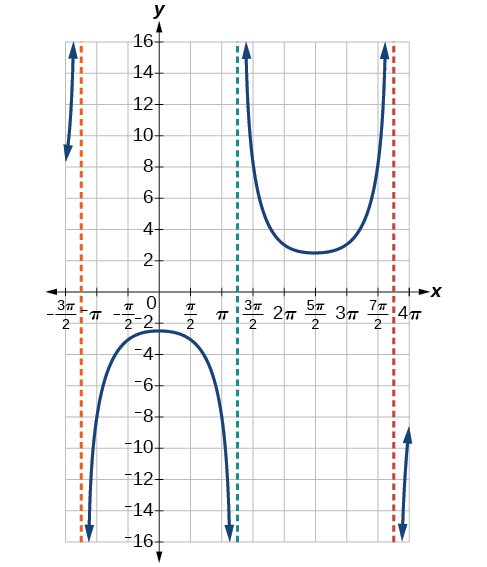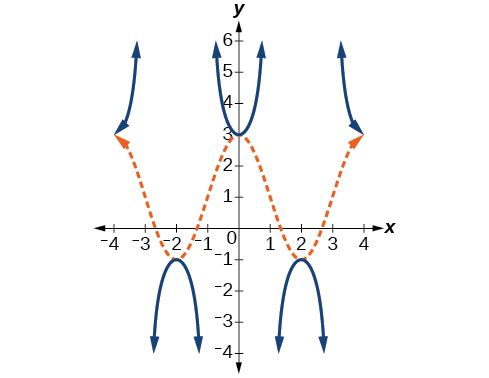8.2 Graphs of the other trigonometric functions  (Page 6/9)

 Page 6 / 9

The domain of $\text{\hspace{0.17em}}\mathrm{csc}\text{\hspace{0.17em}}x\text{\hspace{0.17em}}$ was given to be all $\text{\hspace{0.17em}}x\text{\hspace{0.17em}}$ such that $\text{\hspace{0.17em}}x\ne k\pi \text{\hspace{0.17em}}$ for any integer $\text{\hspace{0.17em}}k.\text{\hspace{0.17em}}$ Would the domain of $\text{\hspace{0.17em}}y=A\mathrm{csc}\left(Bx-C\right)+D\text{\hspace{0.17em}}\text{be}\text{\hspace{0.17em}}x\ne \frac{C+k\pi }{B}?$

Yes. The excluded points of the domain follow the vertical asymptotes. Their locations show the horizontal shift and compression or expansion implied by the transformation to the original function’s input.

Given a function of the form $\text{\hspace{0.17em}}y=A\mathrm{csc}\left(Bx\right),\text{\hspace{0.17em}}$ graph one period.

1. Express the function given in the form $\text{\hspace{0.17em}}y=A\mathrm{csc}\left(Bx\right).$
2. $\text{\hspace{0.17em}}|A|.$
3. Identify $\text{\hspace{0.17em}}B\text{\hspace{0.17em}}$ and determine the period, $\text{\hspace{0.17em}}P=\frac{2\pi }{|B|}.$
4. Draw the graph of $\text{\hspace{0.17em}}y=A\mathrm{sin}\left(Bx\right).$
5. Use the reciprocal relationship between $\text{\hspace{0.17em}}y=\mathrm{sin}\text{\hspace{0.17em}}x\text{\hspace{0.17em}}$ and $\text{\hspace{0.17em}}y=\mathrm{csc}\text{\hspace{0.17em}}x\text{\hspace{0.17em}}$ to draw the graph of $\text{\hspace{0.17em}}y=A\mathrm{csc}\left(Bx\right).$
6. Sketch the asymptotes.
7. Plot any two reference points and draw the graph through these points.

Graphing a variation of the cosecant function

Graph one period of $\text{\hspace{0.17em}}f\left(x\right)=-3\mathrm{csc}\left(4x\right).$

• Step 1. The given function is already written in the general form, $\text{\hspace{0.17em}}y=A\mathrm{csc}\left(Bx\right).$
• Step 2. $\text{\hspace{0.17em}}|A|=|-3|=3,$ so the stretching factor is 3.
• Step 3. $\text{\hspace{0.17em}}B=4,$ so $\text{\hspace{0.17em}}P=\frac{2\pi }{4}=\frac{\pi }{2}.\text{\hspace{0.17em}}$ The period is $\text{\hspace{0.17em}}\frac{\pi }{2}\text{\hspace{0.17em}}$ units.
• Step 4. Sketch the graph of the function $\text{\hspace{0.17em}}g\left(x\right)=-3\mathrm{sin}\left(4x\right).$
• Step 5. Use the reciprocal relationship of the sine and cosecant functions to draw the cosecant function .
• Steps 6–7. Sketch three asymptotes at $\text{\hspace{0.17em}}x=0,\text{\hspace{0.17em}}x=\frac{\pi }{4},\text{\hspace{0.17em}}$ and $\text{\hspace{0.17em}}x=\frac{\pi }{2}.\text{\hspace{0.17em}}$ We can use two reference points, the local maximum at $\text{\hspace{0.17em}}\left(\frac{\pi }{8},-3\right)\text{\hspace{0.17em}}$ and the local minimum at $\text{\hspace{0.17em}}\left(\frac{3\pi }{8},3\right).$ [link] shows the graph.

Graph one period of $\text{\hspace{0.17em}}f\left(x\right)=0.5\mathrm{csc}\left(2x\right).$Given a function of the form $\text{\hspace{0.17em}}f\left(x\right)=A\mathrm{csc}\left(Bx-C\right)+D,\text{\hspace{0.17em}}$ graph one period.

1. Express the function given in the form $\text{\hspace{0.17em}}y=A\mathrm{csc}\left(Bx-C\right)+D.$
2. Identify the stretching/compressing factor, $\text{\hspace{0.17em}}|A|.$
3. Identify $\text{\hspace{0.17em}}B\text{\hspace{0.17em}}$ and determine the period, $\text{\hspace{0.17em}}\frac{2\pi }{|B|}.$
4. Identify $\text{\hspace{0.17em}}C\text{\hspace{0.17em}}$ and determine the phase shift, $\text{\hspace{0.17em}}\frac{C}{B}.$
5. Draw the graph of $\text{\hspace{0.17em}}y=A\mathrm{csc}\left(Bx\right)\text{\hspace{0.17em}}$ but shift it to the right by and up by $\text{\hspace{0.17em}}D.$
6. Sketch the vertical asymptotes, which occur at $\text{\hspace{0.17em}}x=\frac{C}{B}+\frac{\pi }{|B|}k,$ where $\text{\hspace{0.17em}}k\text{\hspace{0.17em}}$ is an integer.

Graphing a vertically stretched, horizontally compressed, and vertically shifted cosecant

Sketch a graph of $\text{\hspace{0.17em}}y=2\mathrm{csc}\left(\frac{\pi }{2}x\right)+1.\text{\hspace{0.17em}}$ What are the domain and range of this function?

• Step 1. Express the function given in the form $\text{\hspace{0.17em}}y=2\mathrm{csc}\left(\frac{\pi }{2}x\right)+1.$
• Step 2. Identify the stretching/compressing factor, $\text{\hspace{0.17em}}|A|=2.$
• Step 3. The period is $\text{\hspace{0.17em}}\frac{2\pi }{|B|}=\frac{2\pi }{\frac{\pi }{2}}=\frac{2\pi }{1}\cdot \frac{2}{\pi }=4.$
• Step 4. The phase shift is $\text{\hspace{0.17em}}\frac{0}{\frac{\pi }{2}}=0.$
• Step 5. Draw the graph of $\text{\hspace{0.17em}}y=A\mathrm{csc}\left(Bx\right)\text{\hspace{0.17em}}$ but shift it up $\text{\hspace{0.17em}}D=1.$
• Step 6. Sketch the vertical asymptotes, which occur at $\text{\hspace{0.17em}}x=0,x=2,x=4.$

The graph for this function is shown in [link] .

Given the graph of $\text{\hspace{0.17em}}f\left(x\right)=2\mathrm{cos}\left(\frac{\pi }{2}x\right)+1\text{\hspace{0.17em}}$ shown in [link] , sketch the graph of $\text{\hspace{0.17em}}g\left(x\right)=2\mathrm{sec}\left(\frac{\pi }{2}x\right)+1\text{\hspace{0.17em}}$ on the same axes.Analyzing the graph of y = cot x

The last trigonometric function we need to explore is cotangent    . The cotangent is defined by the reciprocal identity $\text{\hspace{0.17em}}\mathrm{cot}\text{\hspace{0.17em}}x=\frac{1}{\mathrm{tan}\text{\hspace{0.17em}}x}.\text{\hspace{0.17em}}$ Notice that the function is undefined when the tangent function is 0, leading to a vertical asymptote in the graph at $\text{\hspace{0.17em}}0,\pi ,\text{\hspace{0.17em}}$ etc. Since the output of the tangent function is all real numbers, the output of the cotangent function is also all real numbers.

We can graph $\text{\hspace{0.17em}}y=\mathrm{cot}\text{\hspace{0.17em}}x\text{\hspace{0.17em}}$ by observing the graph of the tangent function because these two functions are reciprocals of one another. See [link] . Where the graph of the tangent function decreases, the graph of the cotangent function increases. Where the graph of the tangent function increases, the graph of the cotangent function decreases.

A laser rangefinder is locked on a comet approaching Earth. The distance g(x), in kilometers, of the comet after x days, for x in the interval 0 to 30 days, is given by g(x)=250,000csc(π30x). Graph g(x) on the interval [0, 35]. Evaluate g(5)  and interpret the information. What is the minimum distance between the comet and Earth? When does this occur? To which constant in the equation does this correspond? Find and discuss the meaning of any vertical asymptotes.
The sequence is {1,-1,1-1.....} has
how can we solve this problem
Sin(A+B) = sinBcosA+cosBsinA
Prove it
Eseka
Eseka
hi
Joel
June needs 45 gallons of punch. 2 different coolers. Bigger cooler is 5 times as large as smaller cooler. How many gallons in each cooler?
7.5 and 37.5
Nando
find the sum of 28th term of the AP 3+10+17+---------
I think you should say "28 terms" instead of "28th term"
Vedant
the 28th term is 175
Nando
192
Kenneth
if sequence sn is a such that sn>0 for all n and lim sn=0than prove that lim (s1 s2............ sn) ke hole power n =n
write down the polynomial function with root 1/3,2,-3 with solution
if A and B are subspaces of V prove that (A+B)/B=A/(A-B)
write down the value of each of the following in surd form a)cos(-65°) b)sin(-180°)c)tan(225°)d)tan(135°)
Prove that (sinA/1-cosA - 1-cosA/sinA) (cosA/1-sinA - 1-sinA/cosA) = 4
what is the answer to dividing negative index
In a triangle ABC prove that. (b+c)cosA+(c+a)cosB+(a+b)cisC=a+b+c.
give me the waec 2019 questionsByByByByByByBy RhodesBy